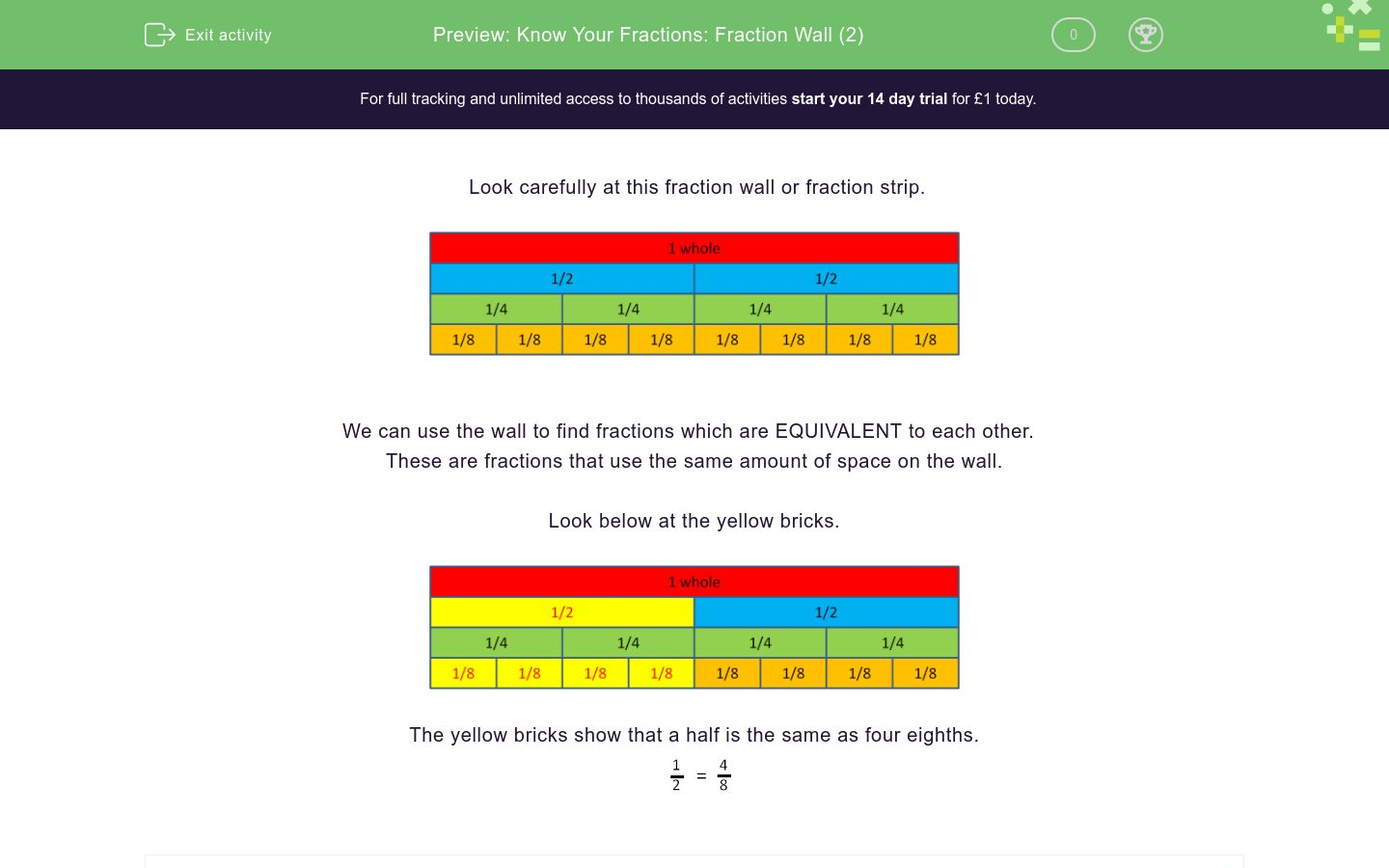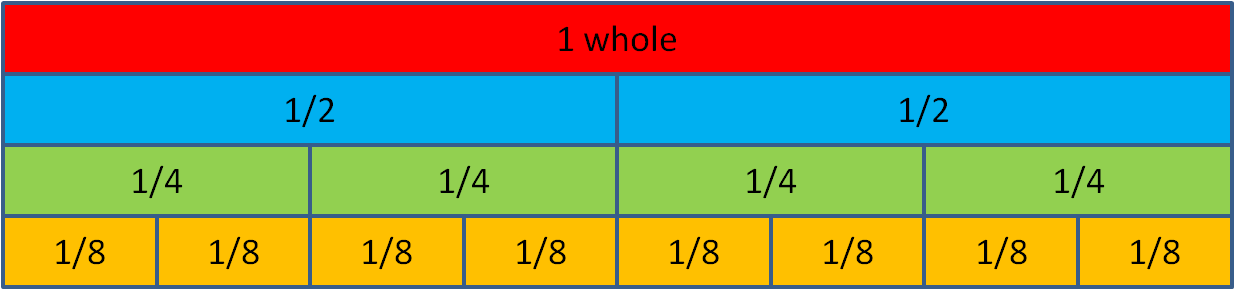# Know Your Fractions: Fraction Wall (2)

In this worksheet, students use a fraction wall to find equivalent fractions.Key stage:  KS 2

Curriculum topic:   Number: Fractions

Curriculum subtopic:   Use Fractions

Difficulty level:### QUESTION 1 of 10

Look carefully at this fraction wall or fraction strip.We can use the wall to find fractions which are EQUIVALENT to each other.

These are fractions that use the same amount of space on the wall.

Look below at the yellow bricks.The yellow bricks show that a half is the same as four eighths.Use the fraction wall to write down how many wholes there are in 8 eighths.Use the fraction wall to write down how many halves there are in 8 eighths.Use the fraction wall to write down how many quarters there are in two halves.Use the fraction wall to write down how many quarters there are in three quarters.Use the fraction wall to write down how many eighths there are in three quarters.Use the fraction wall to write down how many eighths there are in a half.Use the fraction wall to write down how many eighths there are in four quarters.Use the fraction wall to write down how many quarters there are in six eighths.Use the fraction wall to write down how many halves there are in two wholes.Use the fraction wall to write down how many quarters there are in two wholes.• Question 1

Use the fraction wall to write down how many wholes there are in 8 eighths.1
• Question 2

Use the fraction wall to write down how many halves there are in 8 eighths.2
• Question 3

Use the fraction wall to write down how many quarters there are in two halves.4
• Question 4

Use the fraction wall to write down how many quarters there are in three quarters.3
• Question 5

Use the fraction wall to write down how many eighths there are in three quarters.6
• Question 6

Use the fraction wall to write down how many eighths there are in a half.4
• Question 7

Use the fraction wall to write down how many eighths there are in four quarters.8
• Question 8

Use the fraction wall to write down how many quarters there are in six eighths.3
• Question 9

Use the fraction wall to write down how many halves there are in two wholes.4
• Question 10

Use the fraction wall to write down how many quarters there are in two wholes.8
---- OR ----

Sign up for a £1 trial so you can track and measure your child's progress on this activity.

### What is EdPlace?

We're your National Curriculum aligned online education content provider helping each child succeed in English, maths and science from year 1 to GCSE. With an EdPlace account you’ll be able to track and measure progress, helping each child achieve their best. We build confidence and attainment by personalising each child’s learning at a level that suits them.

Get started